Courses

Test: Single Correct MCQs: Compounds Containing Nitrogen | JEE Advanced

23 Questions MCQ Test Class 12 Chemistry 35 Years JEE Mains &Advance Past yr Paper | Test: Single Correct MCQs: Compounds Containing Nitrogen | JEE Advanced

Description
Attempt Test: Single Correct MCQs: Compounds Containing Nitrogen | JEE Advanced | 23 questions in 50 minutes | Mock test for JEE preparation | Free important questions MCQ to study Class 12 Chemistry 35 Years JEE Mains &Advance Past yr Paper for JEE Exam | Download free PDF with solutions
QUESTION: 1

The compound which on reaction with aqueous nitrous acid at low temperature produces an oily nitrosamine is

Solution:

NOTE : Secondary amines (aliphatic as well as aromatic) react with nitrous acid to form Nnitrosoamines.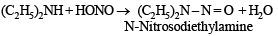QUESTION: 2

Acetamide is treated separately with the following reagents. Which one of these would give methylamine?

Solution: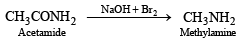QUESTION: 3

Carbylamine test is performed in alcoholic KOH by heating a mixture of :

Solution:

NOTE : Only primary aliphatic and aromatic amines give this test.

CHX3 + RNH2 + 3KOH → RNC + 3 KX + 3H2O

QUESTION: 4

The compound that is most reactive towards electrophilic nitration is :

Solution:

TIPS/Formulae : Toluene has electron-donating methyl group. Hence reacts fastest while others have either electron withdrawing groups (i.e. – COOH or – NO2 etc.) or no substituent.

QUESTION: 5

If two compounds have the same empirical formula but different molecular fomulae they must have

Solution:

TIPS/Formulae : Empirical Formula = n × Molecular formula
Solution : Since the molecular formula is n times the empirical formula, therefore, different compounds having the same empirical formula must have different molecular weights.

QUESTION: 6

Amongst the following, the most basic compound is :

Solution:

In benzylamine, electron pair on nitrogen is not delocalised due to lack of conjugation; while in all other compounds it is delocalised and hence lesser available for protonation.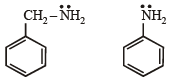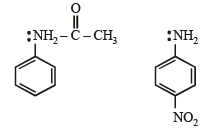QUESTION: 7

The formation of cyanohydrin from a ketone is an example of :

Solution:

TIPS/Formulae : The addition is initiated by the attack of CN group which is a nucleophile.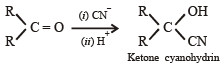QUESTION: 8

Butanonitrile may be prepared by heating :

Solution:

TIPS/Formulae : In the formation of cyanohydrin number of carbon atoms in parent chain increases by one.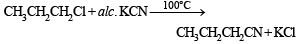QUESTION: 9

In the reaction p-chlorotoluene with KNH2 in liq. NH3,the major product is:

Solution: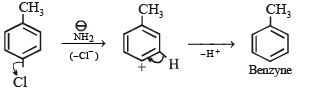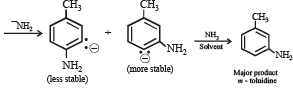QUESTION: 10

The most unlikely representation of resonance structures of p-nitrophenoxide ion is

Solution:

N can't have more than 8 electrons in its valence shell as it does not have any d orbital. In (c), N has 10 electrons.

QUESTION: 11

Among the following, the strongest base is

Solution:

Aliphatic amines are more basic than aromatic amines because in aliphatic amines electron pair on nitrogen is not involved in resonance.

QUESTION: 12

The correct order of basicities of the following compounds is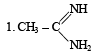2. CH3 - CH2 - NH2

3. (CH3)2NH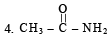Solution: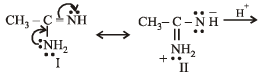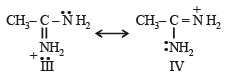The protonated form of II would be III which is more stable because here the contributing structures (III) and (IV) are equivalent.

In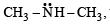the availability of electron pair increases due to the +I effect of two CH3 groups while in  CH3CH2NH2, +I effect of only one ethyl group is operative. In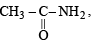the electron availability on nitrogen decreases due to resonance as shown below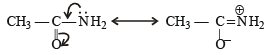Therefore, the order of basic strength would be 1 > 3 > 2 > 4.

QUESTION: 13

Compound 'A' (molecular formula C3H8O) is treated with acidified potassium dichromate to form a product 'B' (molecular formula C3H6O). 'B' forms a shining silver mirror on warming with ammonical silver nitrate. 'B' when treated with an aqueous solution of H2NCONHNH2.HCl and sodium acetate gives a product 'C'. Identify the structure of 'C'.

Solution: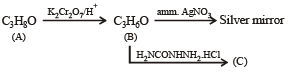NOTE :  Reaction of (B) indicates that it is an aldehyde which thus should be C2H5CHO or CH3CH2CHO, hence C should be CH3CH2CH = NNHCONH2

QUESTION: 14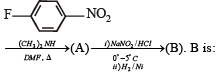Solution: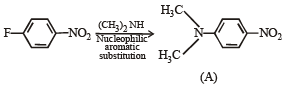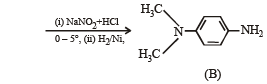QUESTION: 15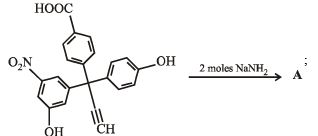The product A will be

Solution:

Two moles of NH2 ions will abstract two moles of most acidic hydrogen out of the four moles of hydrogen present per mole of the acidic compound. The acidic strength is in the order:

–COOH > –OH (phenolic having NO2 group) > –OH (phenolic) > alkynic H.

QUESTION: 16

Benzamide on reaction with POCl3 gives

Solution:

NOTE : POCl3 is a dehydrating agent . Hence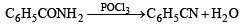QUESTION: 17

The major product obtained when Br2/Fe is treated with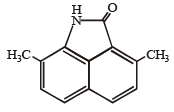Solution:

TIPS/Formulae :– NH is an activating group where as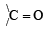group is a deactivating group.
Hence electrophilic substitution will be governed by the ring having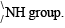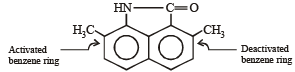QUESTION: 18

In the compound given below the correct order of the acidity of the positions X, Y and Z isSolution:

(i) Position (X) is most acidic due to – COOH group.
(ii) –NH3+ group at position Y is more acidic than at Z because of presence of electron withdrawing – COOH group in close proximity. Hence – NH3+ group at position Z is least acidic.

QUESTION: 19

When benzenesulfonic acid and p-nitrophenol are treated with NaHCO3, the gases released respectively are

Solution:

None of the given options is correct. Benzene sulphonic acid, being stronger acid than carbonic acid, would liberate CO2 when treated with sodium bicarbonate, but p-nitrophenol, being less acidic than carbonic acid, will not liberate CO2.

QUESTION: 20

In the following reaction,

CH3 NH2 + CHCl3+ KOH → Nitrogen containing compound + KCl + H2O.

The nitrogen containing compound is

Solution:

This is an example of carbylamine reaction

CHCl3 + RNH2+ KOH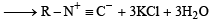QUESTION: 21

The correct stability order of the following resonance structures is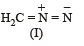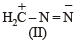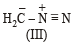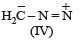Solution: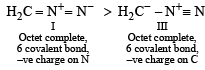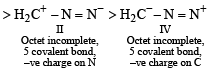QUESTION: 22

The major product of the following reaction is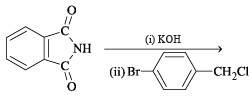Solution: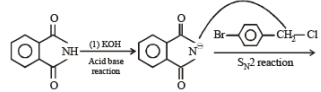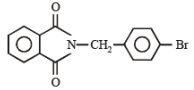QUESTION: 23

Amongst the compounds given, the one that would form a brilliant colored dye on treatment with NaNO2 in dil. HCl followed by addition to an alkaline solution of β-naphthol is

Solution:

Only primary aromatic amines undergo diazotisation followed by coupling.Use Code STAYHOME200 and get INR 200 additional OFF Use Coupon Code

Track your progress, build streaks, highlight & save important lessons and more!

Similar ContentRelated tests